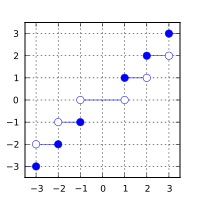/C

# trunc, truncf, truncl

Defined in header `<math.h>`
`float       truncf( float arg );`
(1) (since C99)
`double      trunc( double arg );`
(2) (since C99)
`long double truncl( long double arg );`
(3) (since C99)
Defined in header `<tgmath.h>`
`#define trunc( arg )`
(4) (since C99)
1-3) Computes the nearest integer not greater in magnitude than `arg`.
4) Type-generic macro: If `arg` has type `long double`, `truncl` is called. Otherwise, if `arg` has integer type or the type `double`, `trunc` is called. Otherwise, `truncf` is called.

### Parameters

 arg - floating point value

### Return value

If no errors occur, the nearest integer value not greater in magnitude than `arg` (in other words, `arg` rounded towards zero), is returned.

Return valueArgument

### Error handling

Errors are reported as specified in math_errhandling.

If the implementation supports IEEE floating-point arithmetic (IEC 60559),

• The current rounding mode has no effect.
• If `arg` is ±∞, it is returned, unmodified
• If `arg` is ±0, it is returned, unmodified
• If arg is NaN, NaN is returned

`FE_INEXACT` may be (but isn't required to be) raised when truncating a non-integer finite value.

The largest representable floating-point values are exact integers in all standard floating-point formats, so this function never overflows on its own; however the result may overflow any integer type (including `intmax_t`), when stored in an integer variable.

The implicit conversion from floating-point to integral types also rounds towards zero, but is limited to the values that can be represented by the target type.

### Example

```#include <math.h>
#include <stdio.h>
int main(void)
{
printf("trunc(+2.7) = %+.1f\n", trunc(2.7));
printf("trunc(-2.7) = %+.1f\n", trunc(-2.7));
printf("trunc(-0.0) = %+.1f\n", trunc(-0.0));
printf("trunc(-Inf) = %+f\n",   trunc(-INFINITY));
}```

Possible output:

```trunc(+2.7) = +2.0
trunc(-2.7) = -2.0
trunc(-0.0) = -0.0
trunc(-Inf) = -inf```
• C11 standard (ISO/IEC 9899:2011):
• 7.12.9.8 The trunc functions (p: 253-254)
• 7.25 Type-generic math <tgmath.h> (p: 373-375)
• F.10.6.8 The trunc functions (p: 528)
• C99 standard (ISO/IEC 9899:1999):
• 7.12.9.8 The trunc functions (p: 234)
• 7.22 Type-generic math <tgmath.h> (p: 335-337)
• F.9.6.8 The trunc functions (p: 464)